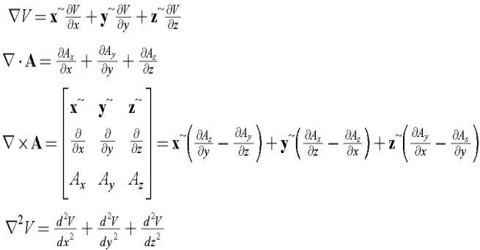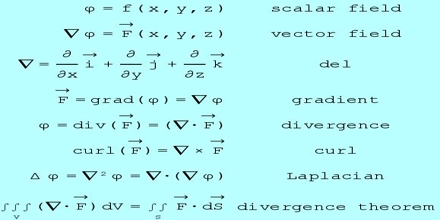Physics

# Vector CalculusVector Calculus

Calculus: In scientific language calculus is a discipline of calculating continuously variable and exceedingly small fraction. In current mathematics it is a vital branch. Vector calculus is a division of mathematics concerned with differentiation and integration of vector fields, mainly in 3-dimensional Euclidean space R3.

Here, the theory of a vector constitutes the mathematical concept of quantities that are characterized not only by a numerical value but also by a direction (for example, force, acceleration, velocity).

Calculus is divided into two parts— (1) Differential calculus and (2) Integral calculus.Differential calculus

• Differential calculus is used to find the function f(x) given ‘s’.
• Differential Calculus deals with the concept of taking a derivative, also called differentiation.
• In differential calculus you find the derivative of a function, which gives you the rate of change of the function at any point, or in other words you get the gradient of the tangent to the curve at a point. Applications include finding maximum and minimum values.

Integral calculus

• Integral calculus is used to find ‘s’ given f(x).
• Integral Calculus deals with taking the Integral of a curve, also called integrating.
• In integral calculus, you do the opposite operation to differentiation, i.e. finding anti-derivative of a function. In other words, integration allows you to take the sum of many infinitesimal elements. Applications include finding area below a graph, volume of revolution of a function, etc.